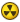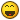## Recommended Posts

Hello, I'm creating a "spam bot" so to speak, if that is what you would like to call it. (All though, that is not really the point of the program)

I have pretty limted expiernce with Auto-it, so I was wondering how to do the following:

Have it choos a random combination of the following letters:

liouysx

And then, what ever combination it chooses, Send("combination").. Need help;)!

##### Share on other sites

Well, here is one way to get the random string:

```\$text = "liouysx"
\$textlen = StringLen(\$text)
for \$i = 1 to \$textlen
\$index = Random(1, \$textlen, 1) ; pick a random character
\$tmp = StringMid(\$text, \$index, 1)  ; store the character in a temporary variable
\$str1 = StringLeft(\$text, \$index - 1) ; split the string, excluding the character
\$str2 = StringRight(\$text, \$textlen - \$index)
\$text = \$tmp & \$str1 & \$str2    ; place the character at the beginning of the string
Next
MsgBox(0, "Random Mix", "Text : " & \$text)```

BlueBearrOddly enough, this is what I do for fun.

##### Share on other sites

Hello, I'm creating a "spam bot" so to speak, if that is what you would like to call it. (All though, that is not really the point of the program)

I have pretty limted expiernce with Auto-it, so I was wondering how to do the following:

Have it choos a random combination of the following letters:

liouysx

And then, what ever combination it chooses, Send("combination").. Need help;)!

Can it be possible to use the same one twice?

```Global \$letter

For \$int = 1 to 7
\$letterNum = Random(1,7,1)

Select
Case \$letternum = 1
\$letter[\$int] = "l"
Case \$letternum = 2
\$letter[\$int] = "i"
Case \$letternum = 3
\$letter[\$int] = "o"
Case \$letternum = 4
\$letter[\$int] = "u"
Case \$letternum = 5
\$letter[\$int] = "s"
Case \$letternum = 6
\$letter[\$int] = "x"
Case \$letternum = 7
\$letter[\$int] = "y"
EndSelect

Next

\$word = \$letter&\$letter&\$letter&\$letter&\$letter&\$letter&\$letter

Msgbox(0, "Random Letters", \$word)```

##### Share on other sites

Hmm if its anything like how the guy hacked the password on national treasure are you sure theres no repeat letters? lol##### Share on other sites

Hmm if its anything like how the guy hacked the password on national treasure are you sure theres no repeat letters? lolIf he doesn't want it to repeat, it can also be done like this

```Global \$letter,\$used=""

For \$int = 1 to 7
\$letterNum = Random(1,7,1)

Select
Case \$letternum = 1
If not StringInStr(\$used, 'l') Then
\$letter[\$int] = "l"
\$used = \$used&"l"
Else
\$int = \$int-1
Endif
Case \$letternum = 2
If not StringInStr(\$used, 's') Then
\$letter[\$int] = "s"
\$used = \$used&"s"
Else
\$int = \$int-1
Endif
Case \$letternum = 3
If not StringInStr(\$used, 'i') Then
\$letter[\$int] = "i"
\$used = \$used&"i"
Else
\$int = \$int-1
Endif
Case \$letternum = 4
If not StringInStr(\$used, 'o') Then
\$letter[\$int] = "o"
\$used = \$used&"o"
Else
\$int = \$int-1
Endif
Case \$letternum = 5
If not StringInStr(\$used, 'y') Then
\$letter[\$int] = "y"
\$used = \$used&"y"
Else
\$int = \$int-1
Endif
Case \$letternum = 6
If not StringInStr(\$used, 'u') Then
\$letter[\$int] = "u"
\$used = \$used&"u"
Else
\$int = \$int-1
Endif
Case \$letternum = 7
If not StringInStr(\$used, 'x') Then
\$letter[\$int] = "x"
\$used = \$used&"x"
Else
\$int = \$int-1
Endif
EndSelect
Next
\$Used = ""
\$word = \$letter&\$letter&\$letter&\$letter&\$letter&\$letter&\$letter

Msgbox(0, "Random Letters", \$word)```

##### Share on other sites

Or much shorter```;~~~~~~~~~~~~~~~~~~~~~~~~~;
;~~ liouysx random generator ;~~
;~~~~~~~~~~~~~~~~~~~~~~~~~;
Global \$comb, \$rand
\$liouysx = StringSplit("l,i,o,u,y,s,x",",")
Do
\$rand = \$liouysx[Round(Random(1,7))]
If StringInStr(\$comb,\$rand) = 0 Then;if the random letter is still not in the combination,it is added to the combination
\$comb = \$comb & \$rand
EndIf
Until StringLen(\$comb) > 6; it makes a string,which contains random liouysx letters(no one is present twice),until there are 7 characters(no more possibilities)
MsgBox(0,"liouysx random generator","Generated liouysx combination: " & \$comb)
;~~~~~~~~~~~~~~~~~~~~~~~~~;
;~~ liouysx random generator ;~~
;~~~~~~~~~~~~~~~~~~~~~~~~~;```

##### Share on other sites

Or much shorter```;~~~~~~~~~~~~~~~~~~~~~~~~~;
;~~ liouysx random generator ;~~
;~~~~~~~~~~~~~~~~~~~~~~~~~;
Global \$comb, \$rand
\$liouysx = StringSplit("l,i,o,u,y,s,x",",")
Do
\$rand = \$liouysx[Round(Random(1,7))]
If StringInStr(\$comb,\$rand) = 0 Then;if the random letter is still not in the combination,it is added to the combination
\$comb = \$comb & \$rand
EndIf
Until StringLen(\$comb) > 6; it makes a string,which contains random liouysx letters(no one is present twice),until there are 7 characters(no more possibilities)
MsgBox(0,"liouysx random generator","Generated liouysx combination: " & \$comb)
;~~~~~~~~~~~~~~~~~~~~~~~~~;
;~~ liouysx random generator ;~~
;~~~~~~~~~~~~~~~~~~~~~~~~~;```
lol well size doesn't matterEdited by Paulie

##### Share on other sites

lol well size doesn't matterheheSorry for my crazy scripting,but it took me as much time to find that code as you made all your replies on this topicThanks a bunch:)

## Create an account

Register a new account

• ### Recently Browsing   0 members

×

• Wiki

• Back

• #### Beta

• Git
• FAQ
• Our Picks
×
• Create New...